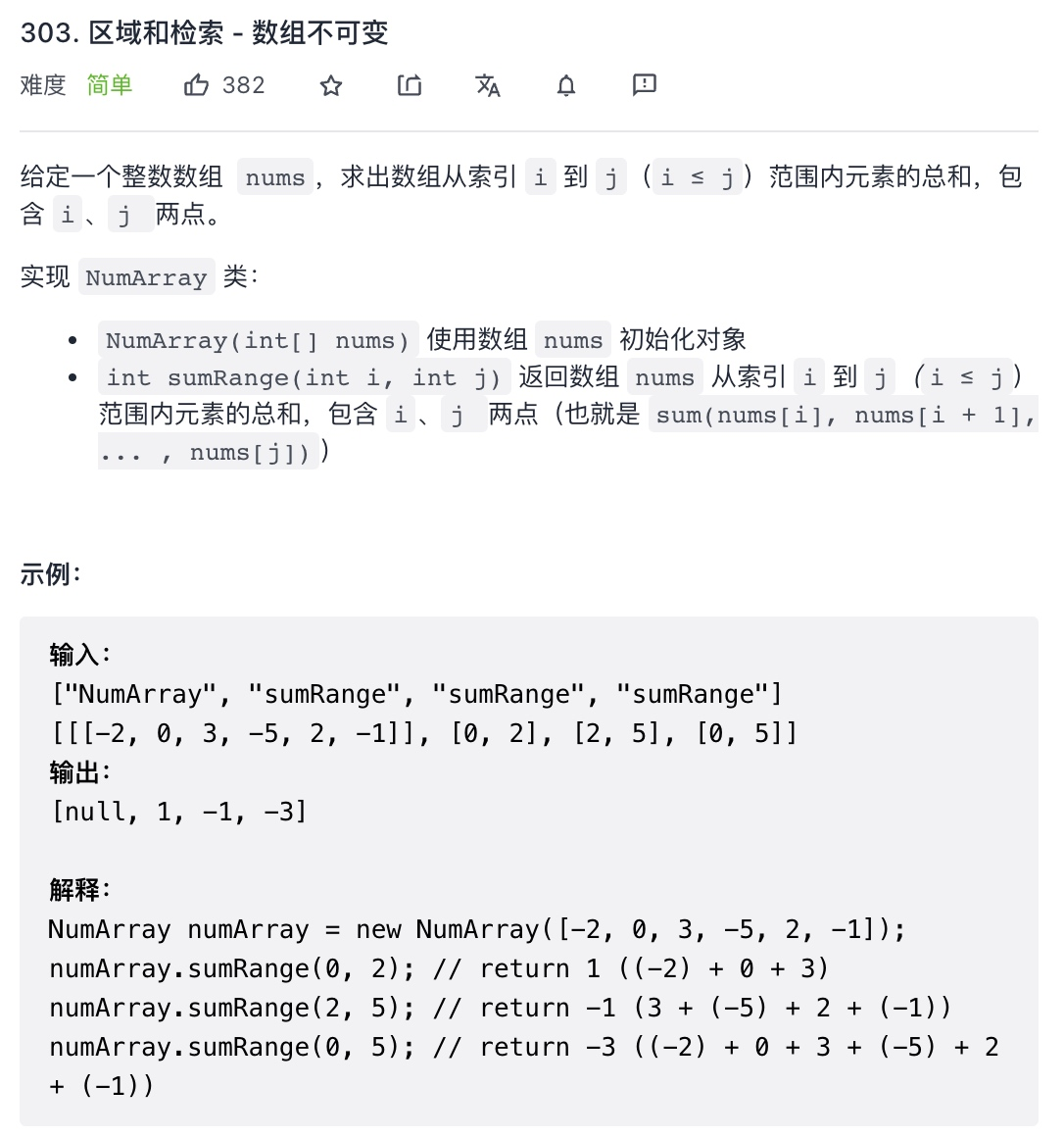# 数组-前缀和技巧

943℃## 解题思路一：遍历数组

``````
class NumArray {
private int[] nums;
public NumArray(int[] nums) {
this.nums = nums;
}
public int sumRange(int left, int right) {
int sum = 0;
for (int i = left; i <= right; i++) {
sum += nums[i];
}
return sum;
}
}

``````

## 解题思路二：使用前缀和技巧

….

``````class NumArray {
private int[] perSum;

public NumArray(int[] nums) {
this.perSum = new int[nums.length +1];
for(int i = 1; i< this.perSum.length; i++ ){
perSum[i] = perSum[i-1] + nums[i-1];
}
}

public int sumRange(int left, int right) {
return perSum[right+1] - perSum[left];
}
}

``````